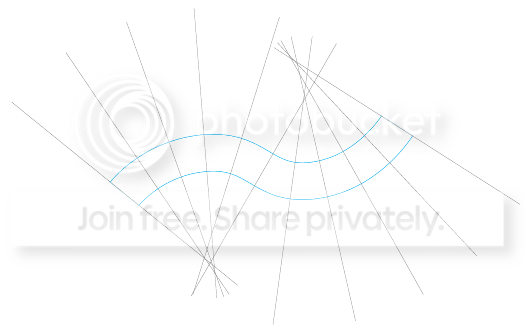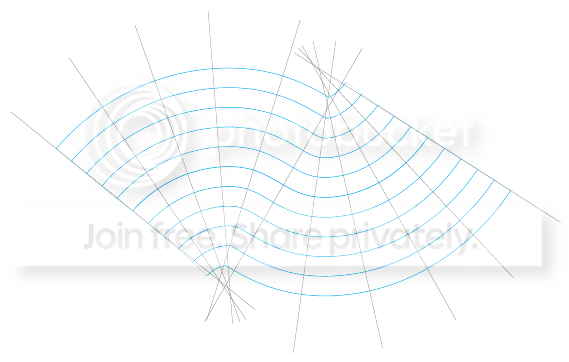# normal coordinate system in tikz using parallel curves

I have a wavey looking curve and would like to show the corresponding normal coordinate system, which is defined by arclength along the curve and (signed) distance from the curve. So there should be lines normal to the curve (for arclength) and curves parallel to the original curve (for distance from the curve).

I am using the solution from Easy curves in TikZ to draw my wavey curve and How to draw tangent line of an arbitrary point on a path in TikZ to get the normal lines. I can draw a single parallel line using double distance=1cm from How to raise a generic curve. Problem with \pgfdeclaredecoration, but I'm not sure how to get a whole set of parallel lines.

Eventually I would also like nodes that label the lines/curves s=1, s=2, ..., r=1, r=2,...,r=0,r=-1,...

Here is my current code:

\documentclass[10pt]{amsart}
\usepackage{tikz}
\usetikzlibrary{decorations.markings}

\tikzstyle{tangent}=[decoration={markings,% switch on markings
mark= at position #1 with {
\coordinate (tangent point-\pgfkeysvalueof{/pgf/decoration/mark info/sequence number}) at (0pt,0pt);
\coordinate (tangent unit vector-\pgfkeysvalueof{/pgf/decoration/mark info/sequence number}) at (1,0pt);
\coordinate (tangent orthogonal unit vector-\pgfkeysvalueof{/pgf/decoration/mark info/sequence number}) at (0pt,1); }}, postaction=decorate]

\tikzstyle{use tangent}=[
shift=(tangent point-#1),
x=(tangent unit vector-#1),
y=(tangent orthogonal unit vector-#1)]

\tikzset{forcedist/.style={decorate, decoration={markings,
mark=between positions 0 and 1 step 0.0999 with {\draw[<-] (0,-#1) -- (0,#1); }}}}%Here
\tikzset{use tangent/.default=1}

\begin{document}
\begin{figure}
\begin{tikzpicture}[scale=.75]
\draw [cyan, double distance = 1cm, tangent=0.001,tangent=0.1,tangent=0.2,tangent=0.3,tangent=0.4,tangent=0.5,tangent=0.6,tangent=0.7,tangent=0.8,tangent=0.9,tangent=0.999] plot [smooth, tension=1] coordinates { (0,.5) (3,2) (7,1) (10,3)};
\foreach \i in {1,...,11} { \draw [very thin,gray,use tangent=\i] (0,-4) -- (0,4); }
\end{tikzpicture}
\end{figure}
\end{document}• Do you also want the curve to scale when increasing and decreasing in +ve and -ve directions? Or is it going to be a shift all the way? Nov 16, 2012 at 9:27
• I'm not sure I understand what you mean by scaling and what "ve" refers to, but I'd like the curves to be constant distances apart, not just a shift up and to the side. Nov 16, 2012 at 16:29
• I meant positive and negative, constant distances apart alright but do they grow as in your example? Nov 16, 2012 at 16:35
• Do you mean do the lengths of the curves grow? If so, then sure, whatever requires them to maintain a constant distance. Nov 16, 2012 at 21:27

After reading the manual, "double distance" is just a line with a large width...so here is a hackish way of producing what I wanted:

(using same preamble as above)

\begin{tikzpicture}[scale=.75]
\foreach \i in {5,...,1} {
\draw [cyan, double distance = \i cm, tangent=0.001,tangent=0.1,tangent=0.2,tangent=0.3,tangent=0.4,tangent=0.5,tangent=0.6,tangent=0.7,tangent=0.8,tangent=0.9,tangent=0.999] plot [smooth, tension=1] coordinates { (0,.5) (3,2) (7,1) (10,3)}; }
\draw [cyan, tangent=0.001,tangent=0.1,tangent=0.2,tangent=0.3,tangent=0.4,tangent=0.5,tangent=0.6,tangent=0.7,tangent=0.8,tangent=0.9,tangent=0.999] plot [smooth, tension=1] coordinates { (0,.5) (3,2) (7,1) (10,3)};
\foreach \i in {1,...,11} { \draw [very thin,gray,use tangent=\i] (0,-4) -- (0,4); }
\end{tikzpicture}Please feel free to submit other answers, as I feel there should be a much more elegant way of doing this!!